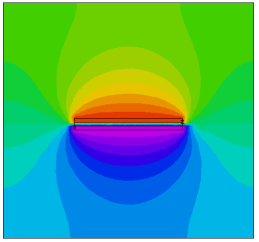﻿ Sample Problems > Applications > Electricity > 3d_capacitor_check

# 3d_capacitor_check

Navigation:  Sample Problems > Applications > Electricity >

# 3d_capacitor_check{  3D_CAPACITOR_CHECK.PDE

This problem shows a parallel-plate capacitor, and compares the computed

capacitance to the ideal value.

}

 TITLE '3D Capacitor validation'   COORDINATES  CARTESIAN3   SELECT  { rename the axes } alias(x) = "X(mm)"     alias(y) = "Y(mm)" alias(z) = "Z(mm)"  { paint all contours }  PAINTED                 VARIABLES  V   DEFINITIONS  Kmetal=1e6  Kdiel = 88   { Water @ 0 C }  Kair=1K = Kair     { default K to Kair - this will change in some layers/regions }

V0 = 0

V1 = 1

X0 = 2    Xwid = 3    X1 = X0+Xwid    X2 = X1+X0      Xc = X2/2

Y0 = 2    Ywid = 3    Y1 = Y0+Ywid    Y2 = Y1+Y0      Yc = Y2/2

Z0 = 3    Zdist=0.1   Zthick=0.1      Zc = Z0+Zdist/2

Eps0 = 8.854e-12                     { Farads/M }

Eps0mm = 0.001*Eps0                   { Farads/mm }

W = integral(0.5*K*eps0mm*grad(V)^2) { Stored Energy }

C = 1.0e6*2*W/(V1-V0)^2               { Capacitance in microFarads }

C0 = 1.0e6*Kdiel*eps0mm*Xwid*Ywid/Zdist

EQUATIONS

EXTRUSION

SURFACE   "Bottom"                    Z=0

LAYER   "Bottom Air"

SURFACE   "Bottom Air - Metal"        Z=Z0-Zthick

LAYER   "Bottom Metal"

SURFACE   "Bottom Metal - Dielectric" Z=Z0

LAYER   "Dielectric"

SURFACE   "Top Metal - Dielectric"    Z=Z0+Zdist

LAYER   "Top Metal"

SURFACE   "Top Metal - Air"           Z=Z0+Zdist+Zthick

LAYER   "Top Air"

SURFACE   "Top"                       Z=Z0+Zthick+Zdist+Zthick+Z0

BOUNDARIES

SURFACE "Bottom" natural(V)=0

SURFACE "Top" natural(V)=0

REGION 1 { this is the outer boundary of the system }

START(0,0)

LINE TO (X2,0) TO (X2,Y2) TO(0,Y2) to close

LIMITED REGION 2 { plates and dielectric }

SURFACE "Bottom Air - Metal"       VALUE(V)=V0
SURFACE "Bottom Metal - Dielectric" VALUE(V)=V0
SURFACE "Top Metal - Dielectric"   VALUE(V)=V1
SURFACE "Top Metal - Air"           VALUE(V)=V1
LAYER "Bottom Metal" K = Kmetal

LAYER "Dielectric" K = Kdiel

LAYER "Top Metal" K = Kmetal

START(X0,Y0)

LAYER "Bottom Metal" VALUE(V)=V0

LAYER "Top Metal" VALUE(V)=V1

LINE TO (X1,Y0) TO (X1,Y1) TO (X0,Y1) to close

MONITORS

CONTOUR(V) ON Y=Yc

REPORT(C) as "Capacitance(uF)"

REPORT(C0) as "Cideal(uF)"

ZOOM(X0-Zthick,Z0-2*Zthick, 5*Zthick,5*Zthick)

PLOTS

CONTOUR(V) ON X=Xc

REPORT(C) as "Capacitance(uF)"

REPORT(C0) as "Cideal(uF)"

CONTOUR(V) ON Y=Yc

REPORT(C) as "Capacitance(uF)"

REPORT(C0) as "Cideal(uF)"

CONTOUR(V) ON  Z=Zc

REPORT(C) as "Capacitance(uF)"

REPORT(C0) as "Cideal(uF)"

CONTOUR(V) ON Y=Yc

ZOOM(X0-Zthick,Z0-2*Zthick, 5*Zthick,5*Zthick)

GRID(X,Z) ON Y=Yc

GRID(X,Y) ON Z=Zc

CONTOUR(log10(K)) ON Y=Yc PAINTED as "Material"

SUMMARY

REPORT(C) as "Capacitance(uF)"

REPORT(C0) as "Cideal(uF)"

REPORT(W) as "Stored Energy"

END